Function Repository Resource:

# GraphMerge

Merge two graphs, linking them at a desired set of vertices

Contributed by: Simon Fischer
 ResourceFunction["GraphMerge"][g1,g2,idx,v] merges two graphs g1 and g2, connecting them at the vertices listed in idx, and using the label v to label the vertices of g2. ResourceFunction["GraphMerge"][g1,g2,dockingIndices] uses the string "v" as a label.

## Details

The ResourceFunction["GraphMerge"] function allows the merging of two graphs g1 and g2 as long as they have a set of common vertex labels that is not empty. If they do not have any vertex labels in common, the output will be two separate graphs as there are no common docking indices.
After merging, the vertex labels of g2 are relabeled to avoid further clashes, using the label specified in v. The string "v" is used by default, with the subscripts running from the number of docking indices to the number of vertices in g2.

## Examples

### Basic Examples (2)

Merge a Petersen graph and a complete graph at three of their vertices:

 In:=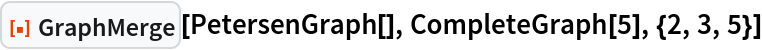Out=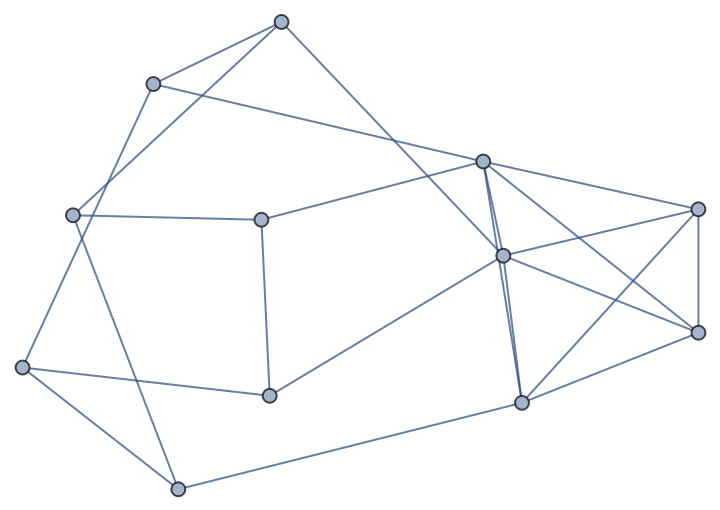Display the vertex labels:

 In:=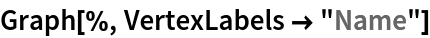Out=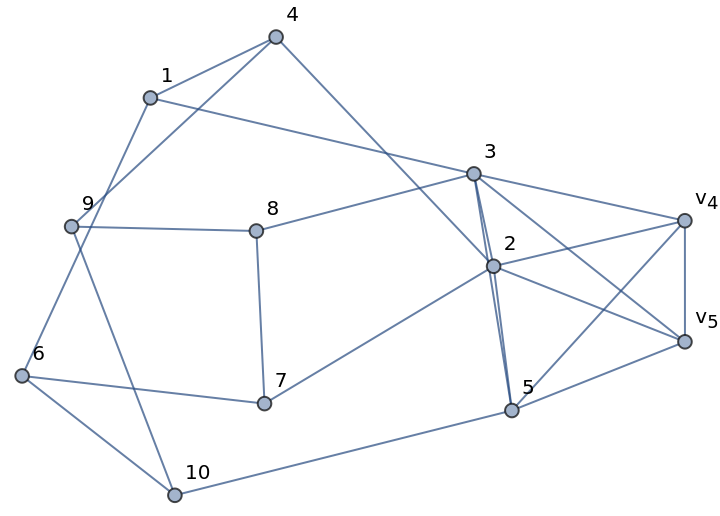### Scope (3)

Merge two grid graphs of different sizes:

 In:=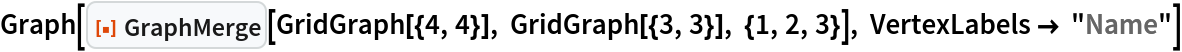Out=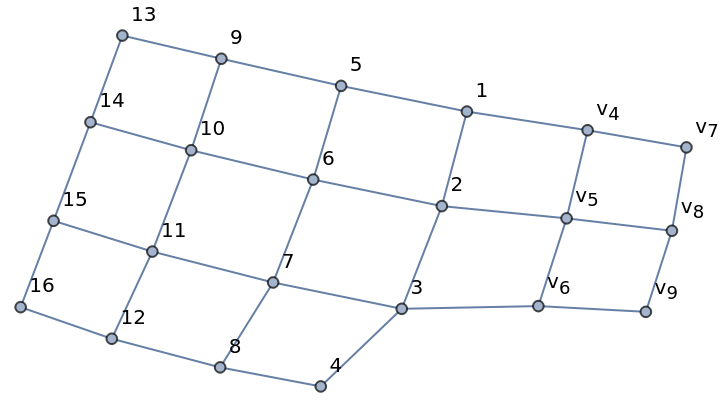Merge two grid graphs of different dimensions:

 In:=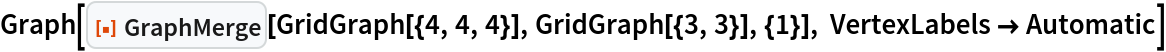Out=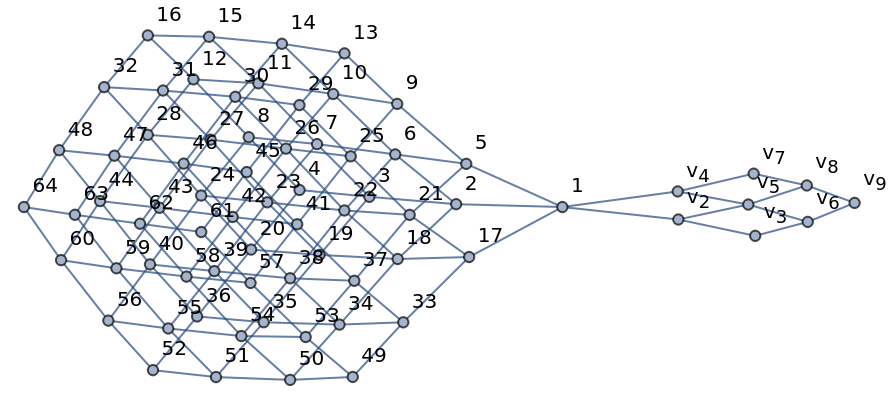Merge a directed and an undirected graph:

 In:=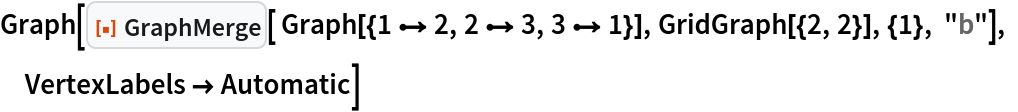Out=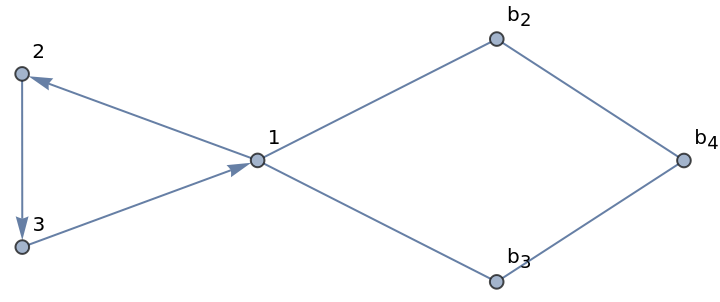### Applications (2)

A grid graph:

 In:=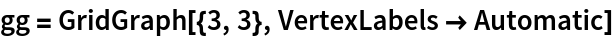Out=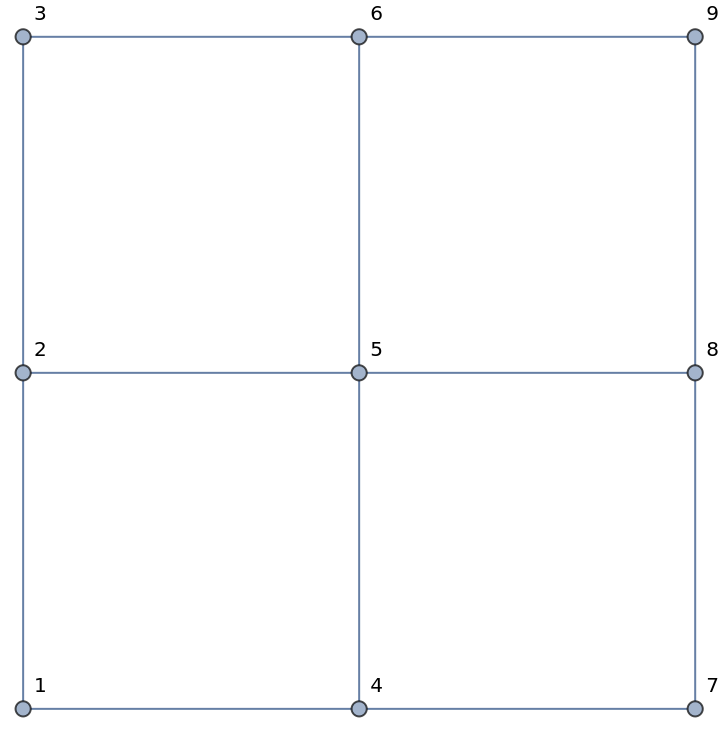Iteratively merge copies of the grid graph:

 In:=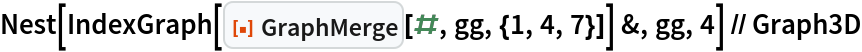Out=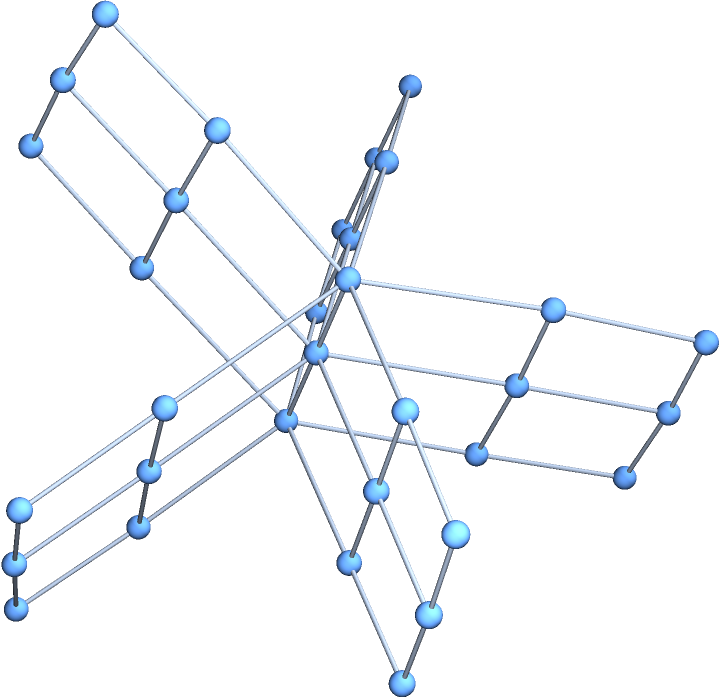### Possible Issues (1)

GraphMerge does not preserve the spatial appearance of the component graphs:

 In:=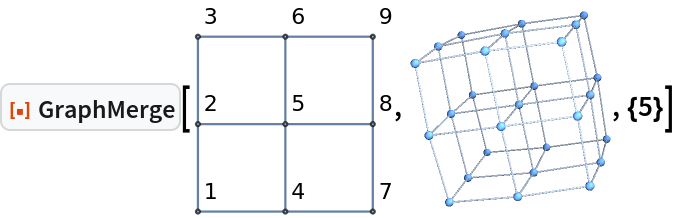Out=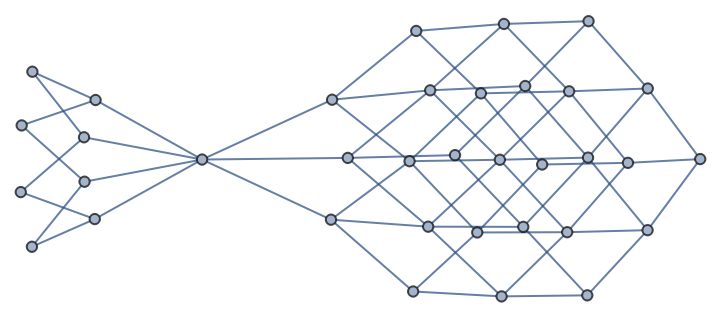Simon Fischer

## Version History

• 1.0.0 – 25 July 2022

## Author Notes

If one applies the function recursively, one should make sure to choose unique labels by specifying the arguments vertexName.